V.5 No 2 25 On light aberration4. On possibility to register the absolute angle of light aberration and to reveal the Earth motion relatively the aether When in the beginning of this paper we considered the physical basis of aberration, we pointed that today this effect is registered by way of observation of one and the same far star at the opposite locations of the Earth in its orbit. This is because “we can determine the angle of deviation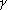(angle of aberration - Authors) only in case if we are able to observe the angle of incidence… In the opposite case we have to change the speed of inlet (in Fig. 7 - Authors) by the known value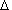u and to measure the corresponding change of direction. The simplest way is to change the direction of velocity by 180o, thenu = 2u  and= 2” [4, p. 192]. Consider the possibility to measure the absolute speed of the Earth by this method of partial inversion of observer’s velocity. Suppose for it that the reference frame accompanying the Earth moves onward with the velocity v0 and at the same time it makes a plane-parallel motion along a slightly elliptical orbit with the velocity vE, as shown in Fig. 8.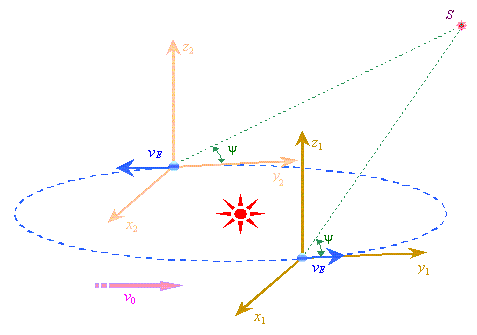Fig. 8. An onward motion with the velocity v0 of reference frame accompanying the Earth, which at the same time makes a plane-parallel motion along a slightly elliptical orbit with velocity vE
 Furthermore, suppose that, firstly, we can neglect the size of the Earth orbit in comparison with the distance to the stationary source. And secondly, we will presume that we make our measurements in the near-inertial regions, where the Earth moves in parallel with the motion of planetary system as such. Under such conditions, the observation angle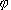and aberration angle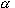in each our measurement will be different with the equal observation angles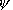of the star S, i.e.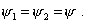(63)
 Then on the grounds of (2) we can write down the relationship in these angles for both measurements. For the fists measurement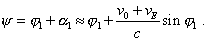(64)
 and for the second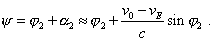(65)
 Subtracting (65) from (64), after transformation we yield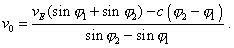(66)
 The expression (66) shows that, using the method of partial inversion of speed, with the help of aberration angle we can determine the absolute speed of Earth motion relatively the aether, though some experimental and calculation difficulties are an obstacle to make it practically. We can mention most hard of them. Firstly, the measurement with this technique would be done in large time intervals; at small difference in1 and2 it causes large error. Secondly, it would be quite hard to put the conditions of experiment to a strong match with the method, in particular to keep the direction of velocity vE strongly coinciding with that of v0 which, additionally, is unknown before the experiments. Thirdly, after this method we would be practically unable to account the peculiar motions of the source. Fourthly, the right part of (66) is a difference of very large values, while the difference in1 and2 is very small. This will cause the inadmissibly large error in computations. Fifthly and the main, with this method we would be able to measure only the projection of velocity v0 onto the orbital plane. But if the orbit was not excited (in case of the Earth orbit this reveals in its small eccentricity), its plane is positioned perpendicularly to the Sun system spatial motion. Due to this, the projection of the velocity v0 onto the orbital plane will be practically zero. This causes equal aberration angles in measurements made conventionally by the method of inverse speed.

Contents: / 18 / 19 / 20 / 21 / 22 / 23 / 24 / 25 / 26 / 27 / 28 /